# Tensorflow 2.0 到底好在哪里？TensorFlow 现在已经不仅是一个单纯的工具包了，而是发展成为了一个平台，在易用性、分布式训练和部署等方面都取得了长足的进步。

TensorFlow 2.0 网站将该项目描述为“端到端开源机器学习平台”。实际上 TensorFlow 已进化成为一个更全面的“工具、库和社区资源生态系统”，可帮助研究人员构建和部署人工智能助力的应用。

TensorFlow 2.0 有四大组成部分：

• TensorFlow 核心，一个用于开发和训练机器学习模型的开源库；

• TensorFlow.js，一个用于在浏览器和 Node.js 上训练和部署模型的 JavaScript 库；

• TensorFlow Lite，一个轻量级库，用于在移动和嵌入式设备上部署模型；

• TensorFlow Extended，一个在大型生产环境中准备数据、训练、验证和部署模型的平台。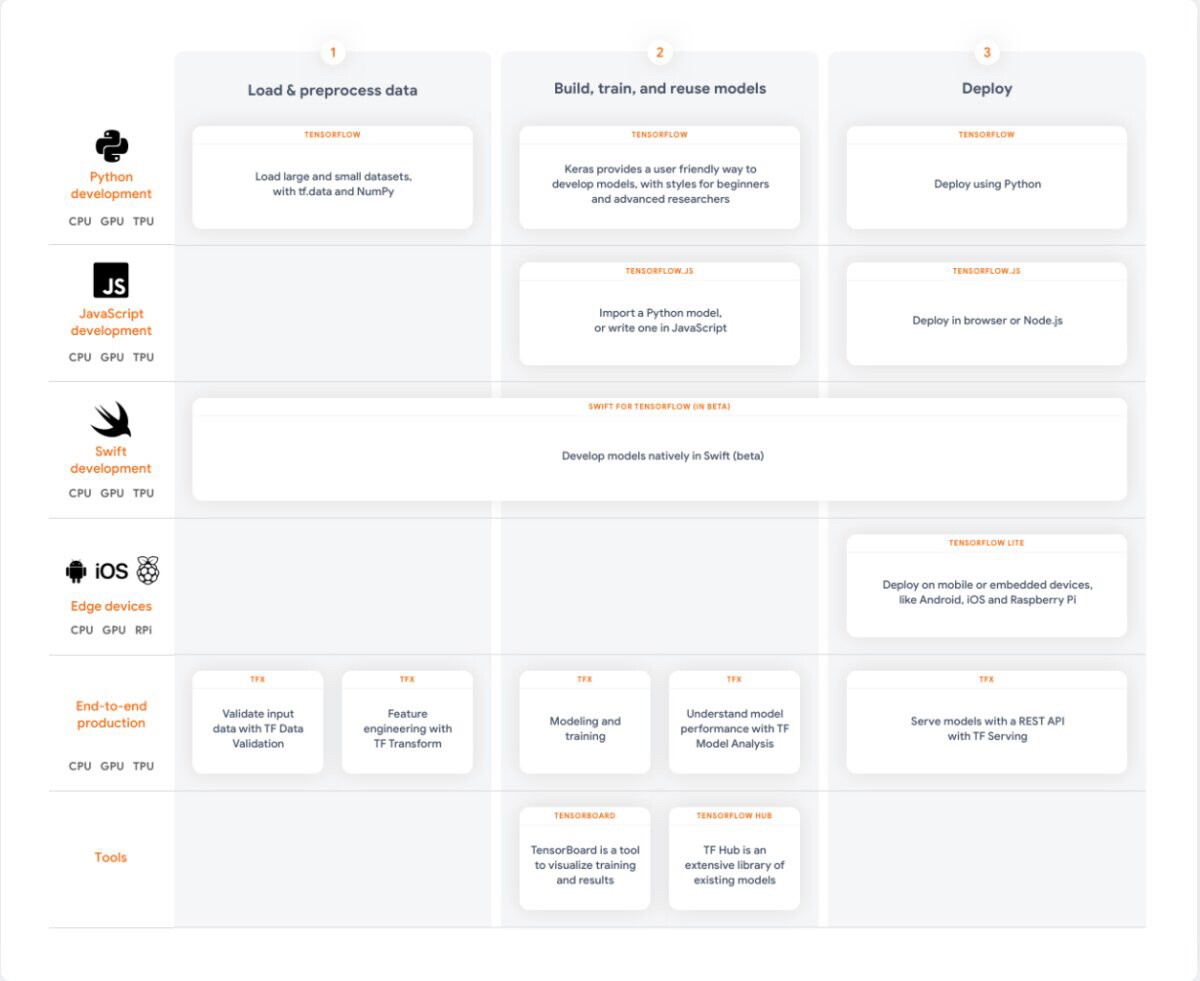TensorFlow 2.0 生态系统包括对 Python、JavaScript 和 Swift 的支持，以及对云、浏览器和边缘设备的部署支持。TensorBoard（可视化）和 TensorFlow Hub（模型库）都是很有用的工具。TensorFlow Extended（TFX）则支持端到端生产流水线。

## TensorFlow 核心

TensorFlow 2.0 的设计重点就是简洁易用，它的新特性包括 Eager Execution、直观的高级 API 以及在任何平台上灵活构建模型等更新。前两个特性值得深入研究。

### Eager Execution

Eager Execution 意味着 TensorFlow 代码被定义后会立即运行，而不是先将节点和边缘添加一个图上，稍后再在一个会话中运行——后者是 TensorFlow 原来使用的模式。例如，TensorFlow r0.10 早期版本的“Hello，World！”脚本如下所示：

\$ python...>>> import tensorflow as tf>>> hello = tf.constant('Hello, TensorFlow!')>>> sess = tf.Session()>>> print(sess.run(hello))Hello, TensorFlow!>>> a = tf.constant(10)>>> b = tf.constant(32)>>> print(sess.run(a + b))42>>> exit()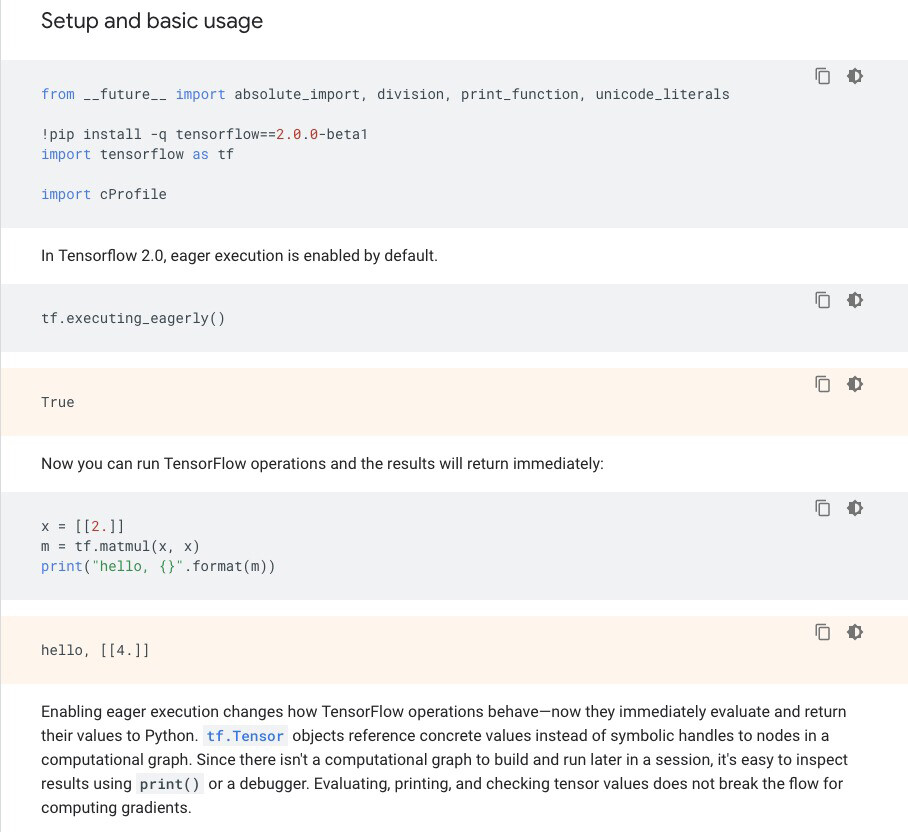TensorFlow 2.0 中的 Eager Execution 示意。这个笔记本可以在谷歌 Colab 中运行，或者在安装好预设的 Jupyter 笔记本中也能运行。

### tf.keras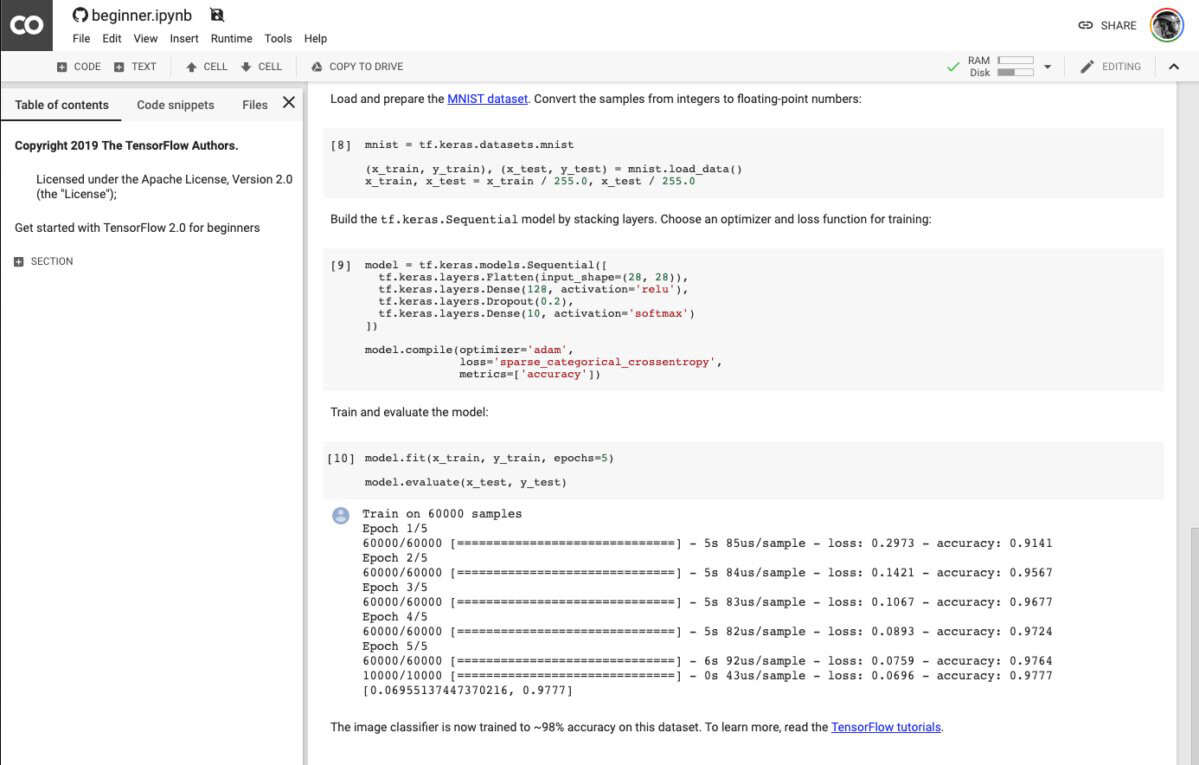## 过渡到 TensorFlow 2.0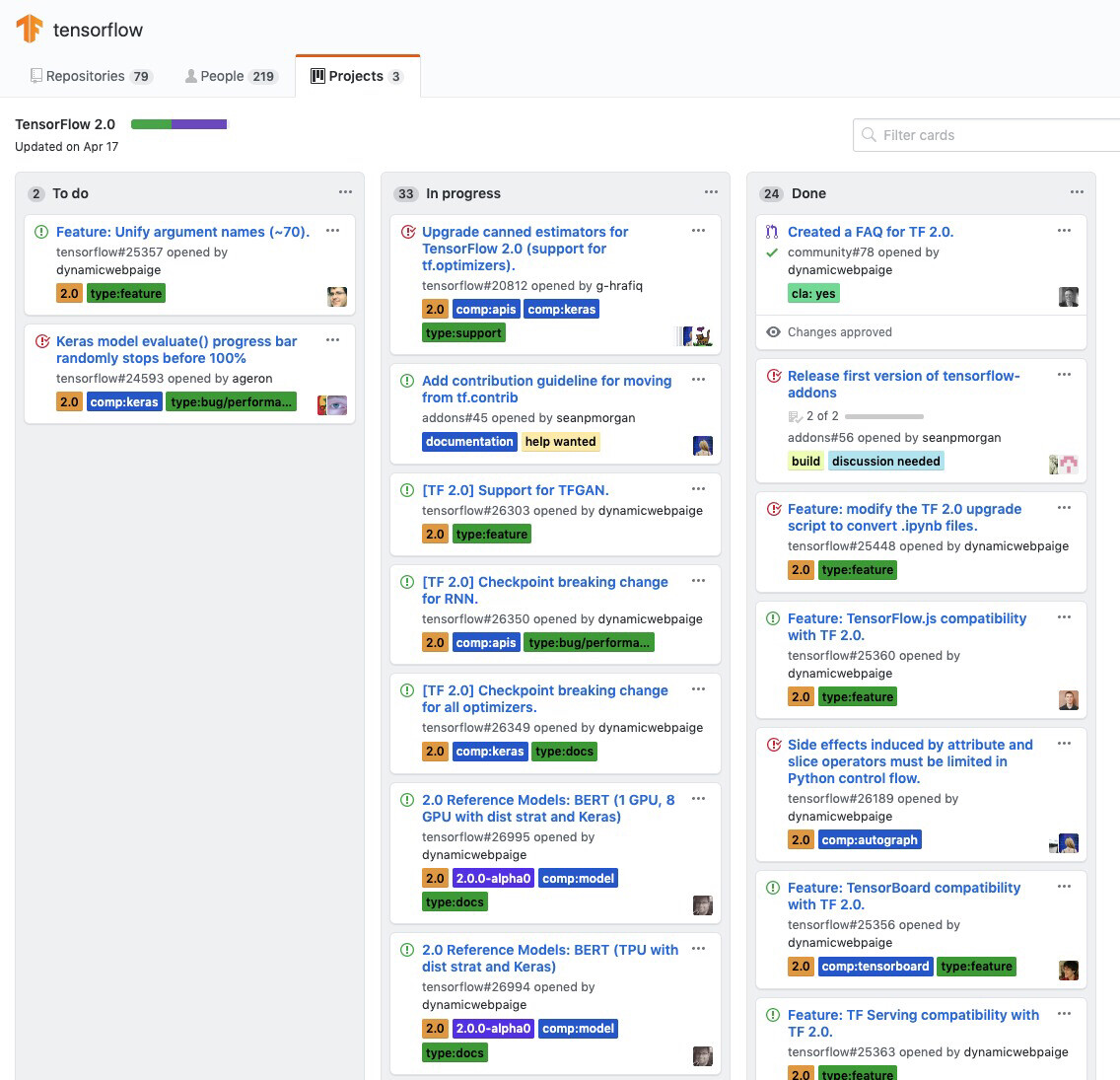## 使用 tf.function

Eager Execution 模式的缺点是可能会损失一些编译和执行流程图的性能。有一种方法可以在不完全关闭 Eager Execution 模式的情况下恢复性能，它就是 tf.function。

## 分布式训练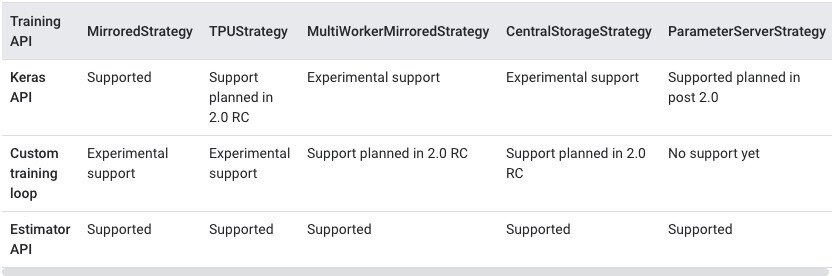TensorFlow 现在支持五种原生分布式策略，TensorFlow 2.0 beta 还对三种训练 API 提供不同级别的支持。

## TensorFlow.js

TensorFlow.js（ https://www.tensorflow.org/js ）是一个用于在 JavaScript 中开发和训练机器学习模型并在浏览器或 Node.js 中部署它们的库。还有一个基于 TensorFlow.js 的高级库 ml5.js（ https://ml5js.org/ ），使用户无需直接面对复杂的张量和优化器。

TensorFlow.js 运行在浏览器中（ https://www.tensorflow.org/js/guide/platform_environment ），支持移动设备和桌面设备。如果你的浏览器支持 WebGL 着色器 API，TensorFlow.js 就可以使用它们并利用 GPU 计算能力，带来相比 CPU 后端多达 100 倍的加速效果。在配备 GPU 的计算机上，TensorFlow.js 演示（ https://www.tensorflow.org/js/demos/ ）在浏览器中运行得非常快。

## TensorFlow Lite

TensorFlow Lite（ https://www.tensorflow.org/lite/ ）是一个用于设备上推断的开源深度学习框架。它目前为 iOS、ARM64 和树莓派构建了模型。

TensorFlow Lite 的两大组件分别是解释器和转换器。解释器用来在许多不同的硬件类型上运行特别针对优化的模型。转换器则将 TensorFlow 模型转换为高效形式供解释器使用，并可引入优化以改善程序体积和性能。预先训练的模型有图像分类、对象检测、智能回复、姿势估计和语义分割等类型。还有用于手势识别、图像分类、对象检测和语音识别的示例应用。

## TensorFlow Extended

TensorFlow Extended（TFX）（ https://www.tensorflow.org/tfx/ ）是用于部署机器学习生产流水线的端到端平台。你训练好一个模型就要考虑使用 TFX 了。其流水线包括数据验证（ https://www.tensorflow.org/tfx/guide/tfdv ）、功能设计（ https://www.tensorflow.org/tfx/transform/get_started ）、建模（ https://www.tensorflow.org/tfx/guide/train ）、模型评估（ https://www.tensorflow.org/tfx/model_analysis/get_started ）、服务推断（ https://www.tensorflow.org/tfx/guide/serving ）以及在线平台、移动原生平台和 JavaScript 平台的部署管理。下图显示了 TFX 流水线的组件是如何组合在一起的。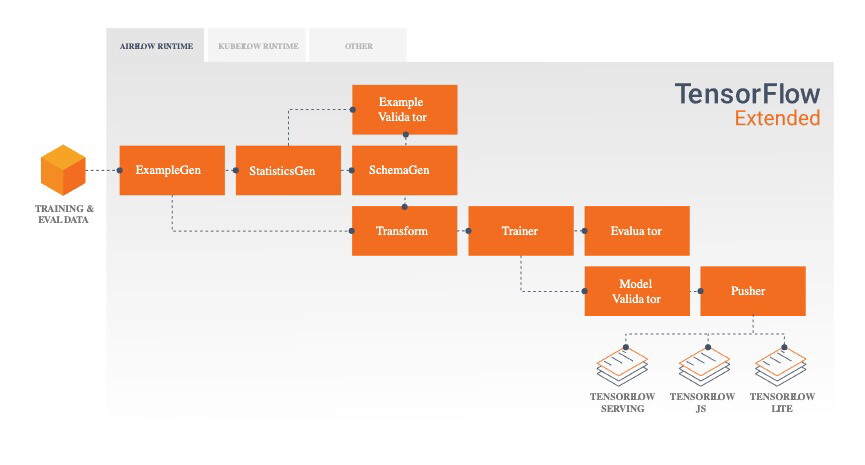TensorFlow Extended 示意图。

## Swift for TensorFlow

Swift for TensorFlow（ https://www.tensorflow.org/swift ）是一个用于深度学习和可微分编程的下一代（并且仍不稳定）平台。它有一个用于训练的高级 API，看起来很像 Python TensorFlow，但它也支持使用 @differentiable 属性自动微分构建到 Swift 编译器的一个 fork 中。Swift for TensorFlow 可以导入和调用 Python 代码，使开发者更容易从 Python TensorFlow 过渡过来。

## TensorFlow 工具